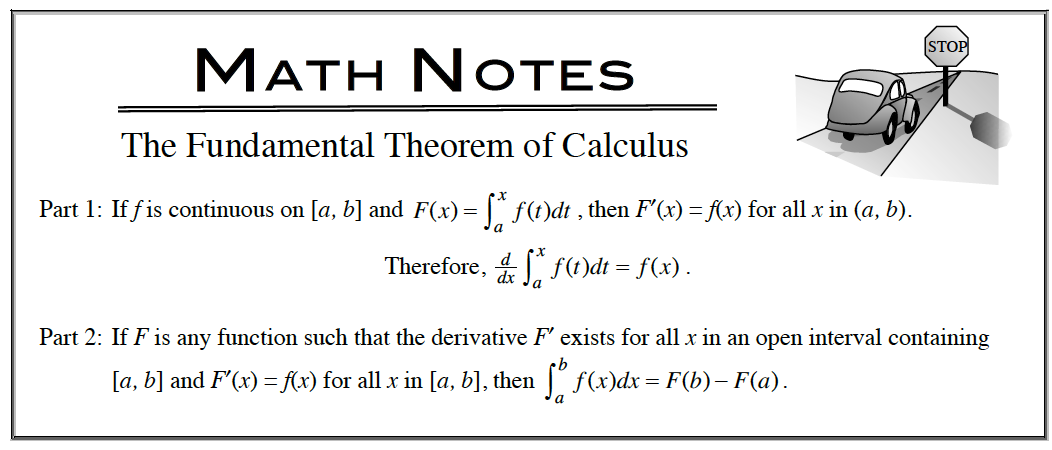Home > APCALC > Chapter 7 > Lesson 7.1.2 > Problem7-21

7-21.

Solve for $y$ if $\frac { d y } { d x }= 3x^{−1/4}− \frac { 7 } { 2 }\sqrt { x }$. Homework Help ✎

$\text{Translation: }y=\int y'(x)dx.$

Solve for y.

The Fundamental Theorem of Calculus, part 1, states that the derivative of an integral is the original function. In this case, you are finding the integral of the derivative. So don't forget to include $+C$.